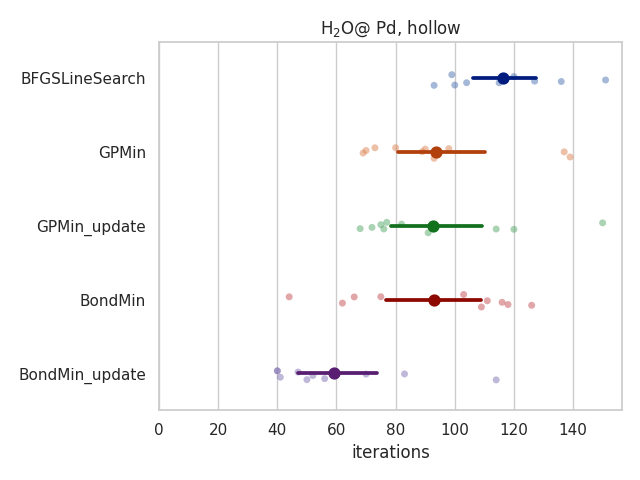# Bondmin optimization algorithm¶

The Paper:

Estefanía Garijo del Río, Sami Kaappa, José A. Garrido Torres, Thomas Bligaard and Karsten W. Jacobsen

Machine Learning with bond information for local structure optimizations in surface science

Preprint

## Brief description¶

This database contains the results of the optimization tests with BondMin and other optimizers (validation and results section of the paper).

The dataset key indicates the test the entry corresponds to. The names of the datasets are the same as in the paper, and a description of the data set can be found in the paper in the data sets section.

A script generating these datasets, as well as the code to run the optimizers can be found at:

https://gitlab.com/egarijo/bondmin

## Key-value pairs¶

key

description

unit

dataset

Data set to which the entry belongs (see paper for more details)

error

Error message raised during the run

n

Number of steps (DFT calculations) needed to optimize the geometry of the system

name

Unique idetifier of the initial structure to the relaxation, in format {formula}_{position(optional)}_{copy_number}

optimizer

Optimizer used for the relaxation

## Example¶

```# creates: bondmin.png
import matplotlib.pyplot as plt
import pandas as pd
import seaborn as sns
from ase.db import connect

sns.set(style='whitegrid')
sns.set_context('notebook')

db = connect('bondmin.db')

selection = 'dataset=MS5,formula=Pd8H2O'
df = {'optimizer': [], 'n': []}

for row in db.select(selection):
if 'hollow' not in row.name:
continue

df['optimizer'].append(row.optimizer)
df['n'].append(row.n)

df = pd.DataFrame(df)

sns.stripplot(x='n', y='optimizer', hue='optimizer',
data=df, alpha=0.5,
zorder=1, palette='deep')

sns.pointplot(x='n', y='optimizer', hue='optimizer',
data=df, linestyles='none',
palette='dark')

plt.xlim(left=0.)
plt.xlabel('iterations')
plt.ylabel('')
plt.title(r'H\${}_2\$O@ Pd, hollow')
plt.tight_layout()
plt.savefig('bondmin.png')
plt.close()
```